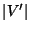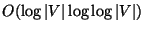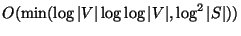Next: MINIMUM FEEDBACK ARC SET Up: Covering and Partitioning Previous: MINIMUM EDGE COLORING   Index

### MINIMUM FEEDBACK VERTEX SET

• INSTANCE: Directed graph.
• SOLUTION: A feedback vertex set, i.e., a subsetsuch that V' contains at least one vertex from every directed cycle in G.
• MEASURE: Cardinality of the feedback vertex set, i.e.,.

• Good News: Approximable withinand .
• Comment: Transformations from MINIMUM VERTEX COVER and MINIMUM FEEDBACK ARC SET . On undirected graphs the problem is APX-complete and approximable within 2, even if the vertices are weighted . The generalized variation in which the input is extended with a subset S of vertices and arcs, and the problem is to find a vertex set that contains at least one vertex from every directed cycle that intersects S, is approximable withinon directed graphs  and within 8 on undirected graphs . All these problems are approximable within 9/4 for planar graphs . The constrained variation in which the input is extended with a positive integer k and a subset S of V, and the problem is to find the feedback vertex set of size k that contains the largest number of vertices from S, is not approximable withinfor some.